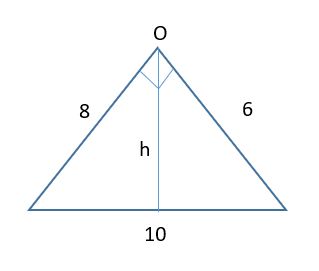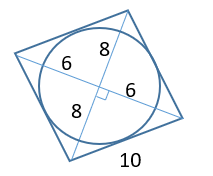# CAT 2020 Question Paper | Quants Slot 1

###### CAT Previous Year Paper | CAT Quants Questions | Question 18

This question is from Geometry. It discusses about a circle that is inscribed in a rhombus. We need to find the ratio of the area of the circle to the area of the rhombus. Geometry is an important topic with lots of weightage in CAT exam. Make sure you master Geometry by practicing a lot of questions.

Question 18 : A circle is inscribed in a rhombus with diagonals 12 cm and 16 cm. The ratio of the area of the circle to the area of the rhombus is

1. $$frac{2π}{15}$ 2. $\frac{6π}{25}$ 3. $\frac{3π}{25}$ 4. $\frac{5π}{18}$ ## Best CAT Online Coaching Try upto 40 hours for free Learn from the best! #### 2IIM : Best Online CAT Coaching. ### Video Explanation ## Best CAT Coaching in Chennai #### CAT Coaching in Chennai - CAT 2022Limited Seats Available - Register Now! ### Explanatory Answer Diagonals of a rhombus are perpendicular bisectors of each other If diagonals are 12 and 16 Half of diagonals are 6 and 8 third side will become 10 = side of rhombus Radius of the circle will be perpendicular to the side of the rhombus Because side of the rhombus is tangent to the circle The triangle is taken by half a diagonal$6) and (8) from the original figureJust equating the areas
$$frac{1}{2}$ × 8 × 6 = $\frac{1}{2}$ × 10 × h h = $\frac{24}{5}$ = same as radius of the circle$r)
Now, area of circle : area of rhombus
π × $$frac{24}{5}$ × $\frac{24}{5}$ : $\frac{1}{2}$ × 12 × 16 6π : 25 The question is "A circle is inscribed in a rhombus with diagonals 12 cm and 16 cm. The ratio of the area of the circle to the area of the rhombus is" ##### Hence, the answer is, "$\frac{6π}{25}$" ###### Best CAT Online Coaching Try upto 40 hours for free Learn from the best! ###### Prepare for CAT 2023 with 2IIM's Daily Preparation Schedule ###### Know all about CAT Exam Syllabus and what to expect in CAT ###### Already have an Account? ###### CAT Coaching in ChennaiCAT 2023 Classroom Batches Starting Now! @Gopalapuram ###### Best CAT Coaching in Chennai Introductory offer of 5000/- Attend a Demo Class ###### Best Indore IPM & Rohtak IPM CoachingSignup and sample 9 full classes for free. Register now! ##### Where is 2IIM located? 2IIM Online CAT Coaching A Fermat Education Initiative, 58/16, Indira Gandhi Street, Kaveri Rangan Nagar, Saligramam, Chennai 600 093 ##### How to reach 2IIM? Mobile:$91) 99626 48484 / 94459 38484
WhatsApp: WhatsApp Now
Email: info@2iim.com